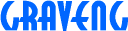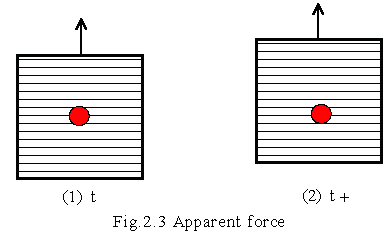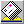2.3 Apparent force

We feel that body is pressed to a seat when a car is accelerated. Such force is called as apparent force, it contains centrifugal force, Coriolis force and so on. The reason why this force is called apparent, it appears by the way of taking a coordinates, seems that the force is not real existence.

Real force must exist if there is apparent force. What is real force? It is said that for example a force pulling a thread is the real force. We would think so, a tension of the thread becomes the real force if a thread is put on the ball and swing. However, the force is centrifugal force too. if centrifugal force is apparent, the tension would be apparent force.

Ultimately, it must be considered that the difference between apparent force and real force isn't being cleared. We can not make light of the difference because of important theme for physics. For instance, suppose that you were beat by someone, if the force was apparent, the someone would not be guilty, but real force, the someone would be a charge of bodily injury. if a judge calls a physicist to the witness, and ask that "which is the force apparent or real?". What does he answer?

What is apparent force? It is explaining as follows in the physics.

"When a coordinates is moving with acceleration 'a' against inertia system, the apparent force is a force to adopt the effect of the acceleration 'a' to set up a Newton's equation of motion in the coordinates. The force must be introduced at exchanging coordinates, is called apparent force not to act against an object as real force. the force is also called as inertia force, the apparent force contains centrifugal force and Coriolis' force."

( Sorry! I'm bad English writer you know, above sentences might not be correct. Things which must be bust are sentences I wrote? )

If real force is "not to act against an object as real", apparent force would be not real force. so centrifugal force is considered that not to act against an object real, but there is an invention as a centrifuge because of centrifugal force act real.

The proposition might contains something wrong (except my bad English), but the first half of sentences has probably something mean. We intend to interpret the apparent force as follows for the time being.

"When a coordinates is moving with acceleration 'a' against inertia system, the apparent force is a force to adopt the effect of the acceleration 'a' to set up a Newton's equation of motion in the coordinates."

If there is apparent in the force, there must be apparent in the velocity as well. The force is considered a subordinate function of velocity with constant mass. To write the apparent velocity like the apparent force proposition as;

"When a coordinates is moving with velocity 'v' against inertia system, the apparent velocity is a velocity to adopt the effect of the velocity 'v' to set up a Newton's equation of motion in the coordinates."

We could not well understand about force, but understandable about velocity. The mean is probably like looking at station from train, it can considered that the station is moving contrary direction of train. The velocity could be considered the same as concept of relative velocity. It could be considered that the meaning of the word of the apparent is substituted for the relative.

we intend to redefine such scientific terms of physics as follows;

apparent velocity ; relative velocity
apparent momentum ; relative momentum = mass * relative velocity
apparent acceleration ; relative acceleration = differential relative velocity
apparent force ; relative force = mass * relative acceleration

If it is interpreted like this, the meaning of apparent force becomes definite. For example, when it is seen from train being accelerated, station has relative acceleration in the opposite direction. Observing from a coordinates based train, it seems that force of relative acceleration is acting against station. However, any object is not pushed by the force of relative acceleration. The apparent force being used in existent physics contains the real force, we must exclude the real force from the apparent force.

Well, What is the real force? We are called non-relative velocity as inertia velocity in "2.2 absolutely velocity". If relative velocity is apparent, non-relative velocity would be the real velocity. We intend to consider the real velocity as inertia velocity, and redefine such scientific terms of physics as follows;

real velocity ; inertia velocity
real momentum ; inertia momentum = mass * inertia velocity
real acceleration ; inertia acceleration = differential inertia velocity
real force ; inertia force = mass * inertia acceleration

"inertia force" of the terms was considered as apparent force in the existent physics. But the force is not apparent, is real. Let's consider this.

Suppose that an elevator is floating in zero gravity space, also an apple is floating in center of the elevator, at the beginning, the apple is rest against the elevator. This mean is that the relative velocity between the apple and the elevator is zero.

Pulling elevator by non-gravitational force, it seems that some force is acting the apple observing from elevator. The force is relative force which occurs by relative acceleration between the elevator and the apple, therefore the force is 'apparent force'.The apple will be having a collision with the wall of elevator soon. When some force will occur between the wall and the apple, the force would be considered that like the force in accelerating car. Is this force 'apparent force'? If the force is relative force, we should approve of that the force of two balls collision is also relative force.

Fundamentally, the apple is not necessary to be inside of elevator, the result is the same as the apple to be outside. It is only phenomenon of two objects collision. The force of pressing against a seat is not necessary to distinguish with real force. The difference between before collision and after is varied of inertia velocity of the apple. In other words, the difference is that energy was added or not.

Apparent force can not change inertia velocity, or energy. Real force can change inertia velocity, or energy.

By the way, acting force against being rest object, which is the force apparent or real? The object was being standstill, it seems not to vary inertia velocity. In general physics, it is considered not necessary energy of this phenomenon. we would think so, the force is apparent? We should be necessary to review about relation force and energy.

Updated 29/Mar/1997redsky@graveng.jp